国际大宗商品市场与国内外股票市场对我国大宗商品市场溢出效应的研究A Study on the Spillover Effects of International Commodity Markets and Domestic and Foreign Stock Markets on China’s Commodity Market

• 全文下载: PDF(1248KB)    PP.976-986   DOI: 10.12677/HJAS.2018.88143
• 下载量: 227  浏览量: 307

With the high internationalization of financial market and the deepening of the financial degree of commodity market, the domestic commodity market will face the impact of many kinds of markets. This paper analyzes the effects of international commodity market and domestic and foreign stock markets on China’s commodity market by using VAR model and DCC-MGARCH model respectively from two angles of mean overflow effect and fluctuation spillover effect. The results show that the mean spillover effect and the volatility spillover effect are obvious to the international stock market and the international commodity market, but the relationship between the domestic stock market and the commodity markets in China is not close enough.

1. 引言

2. 模型构建与数据描述

2.1. 模型构建

2.1.1. 均值方程

VAR模型，即向量自回归模型，通常用于多变量时间序列系统的预测和描述随机扰动对变量系统的动态影响，实际上是向量自回归移动平均(VARMA)的简化  。本文中运用VAR模型对不同金融市场间的均值溢出效应进行分析。均值方程VAR(q)模型的表达式为：

$rccc{i}_{t}={\mu }_{1}+\underset{i=1}{\overset{q}{\sum }}{\alpha }_{1i}rccc{i}_{t-i}+\underset{i=1}{\overset{q}{\sum }}{\beta }_{1i}rcrb{s}_{t-i}+\underset{i=1}{\overset{q}{\sum }}{\theta }_{1i}rcr{b}_{t-i}+\underset{i=1}{\overset{q}{\sum }}{\gamma }_{1i}rmsc{i}_{t-i}+\underset{i=1}{\overset{q}{\sum }}{\eta }_{1i}rs{h}_{t-i}+{\epsilon }_{5t}$

$rcrb{s}_{t}={\mu }_{2}+\underset{i=1}{\overset{q}{\sum }}{\alpha }_{2i}rccc{i}_{t-i}+\underset{i=1}{\overset{q}{\sum }}{\beta }_{2i}rcrb{s}_{t-i}+\underset{i=1}{\overset{q}{\sum }}{\theta }_{3i}rcr{b}_{t-i}+\underset{i=1}{\overset{q}{\sum }}{\gamma }_{3i}rmsc{i}_{t-i}+\underset{i=1}{\overset{q}{\sum }}\eta rs{h}_{t-i}+{\epsilon }_{2t}$

$rcr{b}_{t}={\mu }_{3}+\underset{i=1}{\overset{q}{\sum }}{\alpha }_{3i}rccc{i}_{t-i}+\underset{i=1}{\overset{q}{\sum }}{\beta }_{3i}rcrb{s}_{t-i}+\underset{i=1}{\overset{q}{\sum }}{\theta }_{3i}rcr{b}_{t-i}+\underset{i=1}{\overset{q}{\sum }}{\gamma }_{3i}rmsc{i}_{t-i}+\underset{i=1}{\overset{q}{\sum }}{\eta }_{3i}rs{h}_{t-i}+{\epsilon }_{3t}$

$rmsc{i}_{t}={\mu }_{4}+\underset{i=1}{\overset{q}{\sum }}{\alpha }_{4i}rccc{i}_{t-i}+\underset{i=1}{\overset{q}{\sum }}{\beta }_{4i}rcrb{s}_{t-i}+\underset{i=1}{\overset{q}{\sum }}{\theta }_{4i}rcr{b}_{t-i}+\underset{i=1}{\overset{q}{\sum }}{\gamma }_{4i}rmsc{i}_{t-i}+\underset{i=1}{\overset{q}{\sum }}{\eta }_{4i}rs{h}_{t-i}+{\epsilon }_{4t}$

$rs{h}_{t}={\mu }_{5}+\underset{i=1}{\overset{q}{\sum }}{\alpha }_{5i}rccc{i}_{t-i}+\underset{i=1}{\overset{q}{\sum }}{\beta }_{5i}rcrb{s}_{t-i}+\underset{i=1}{\overset{q}{\sum }}{\theta }_{5i}rcr{b}_{t-i}+\underset{i=1}{\overset{q}{\sum }}{\gamma }_{5i}rmsc{i}_{t-i}+\underset{i=1}{\overset{q}{\sum }}{\eta }_{5i}rs{h}_{t-i}+{\epsilon }_{5t}$

2.1.2. DCC多元变量GARCH模型

DCC-GARCHA模型最早由Engle等人提出，用于研究多变量之间的动态相关性问题  。该模型的参数估计主要分为两步：首先，利用GARCH模型分别对所有变量的收益率序列的波动性进行估计，并获得标准化残差；第二步运用极大似然估计法，对上一步得到的标准化残差序列估计DCC模型的参数和动态相关系数的矩阵  。模型的主要形式如下：

${H}_{t}=E\left({\epsilon }_{t}{{\epsilon }^{\prime }}_{t}|{\Omega }_{t-1}\right)$

${H}_{t}={D}_{t}{R}_{t}{D}_{t}$

t期的误差向量； ${D}_{t}$ 是单一变量GARCH模型估计出的各资产收益率条件标准差构成的对角矩阵，即

${D}_{t}=diag\left({h}_{11,t}^{\frac{1}{2}},\cdots ,{h}_{nn,t}^{\frac{1}{2}}\right)$${h}_{ii,t}$ 为单变量GARCH方程估计出的条件方差。单变量GARCH模型为：

${h}_{ii,t}={c}_{i}+\underset{p=1}{\overset{{p}_{i}}{\sum }}{\alpha }_{i,p}{\epsilon }_{i,t-p}^{2}+\underset{q=1}{\overset{{q}_{i}}{\sum }}{\beta }_{i,q}{h}_{ii,t-q}$

p，q分别为前期残差平方项和前期条件方差的滞后阶数，模型的约束条件为： ${\alpha }_{i,p}\ge 0$${\beta }_{i,p}\ge 0$ ，且 $\underset{p=1}{\overset{{p}_{i}}{\sum }}{\alpha }_{i,p}+\underset{q=1}{\overset{{q}_{i}}{\sum }}{\beta }_{i,p}<1$

DCC模型的形式可以表述为：

$\left\{\begin{array}{l}{R}_{t}={Q}_{t}^{*-1}{Q}_{t}{Q}_{t}^{*-1}\\ {Q}_{t}=\left(1-\underset{m=1}{\overset{M}{\sum }}{\alpha }_{m}-\underset{n=1}{\overset{N}{\sum }}{\beta }_{n}\right)\stackrel{¯}{Q}+\underset{m=1}{\overset{M}{\sum }}{\alpha }_{m}\left({\epsilon }_{t-m}{{\epsilon }^{\prime }}_{t-m}\right)+\underset{n=1}{\overset{N}{\sum }}{\beta }_{n}{Q}_{t-n}\end{array}$

2.2. 数据来源与处理

2.3. 描述性统计

${R}_{t}=\mathrm{ln}\left({P}_{t}\right)-\mathrm{ln}\left({P}_{t-1}\right)$

3. 实证研究结果

3.1. 均值溢出效应估计结果

3.1.1. 均值方程参数估计结果

Table 2. Parameter estimation results of VAR model

3.1.2. 均值溢出效应机制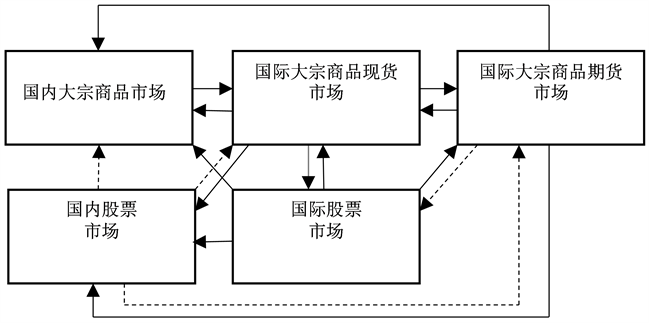注：实线表示正向，虚线表示负向。

Figure 1. Mean spillover effect between different markets

3.2. 波动溢出效应估计结果

3.2.1. DCC-MGARCH模型参数估计结果

3.2.2. 不同市场间的波动溢出效应

1) 国内大宗商品市场与国际大宗商品市场Table 3. The parameter results of the GARCH effect estimationTable 4. DCC model coefficient results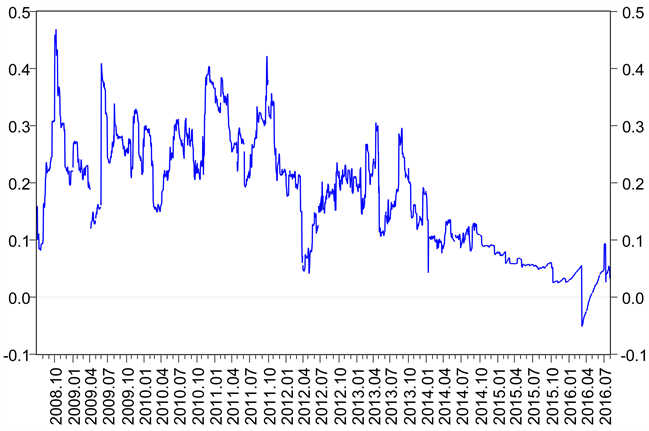Figure 2. Domestic commodity market and international bulk commodity spot market

2) 国内大宗商品市场与国际股票市场

3) 国内大宗商品市场与国内股票市场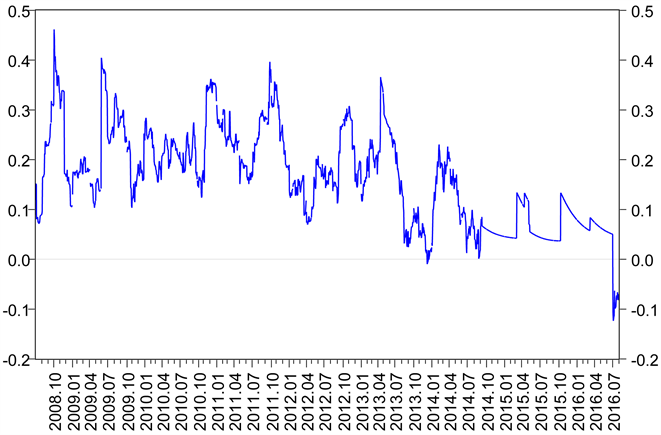Figure 3. Domestic commodity market and international commodity futures market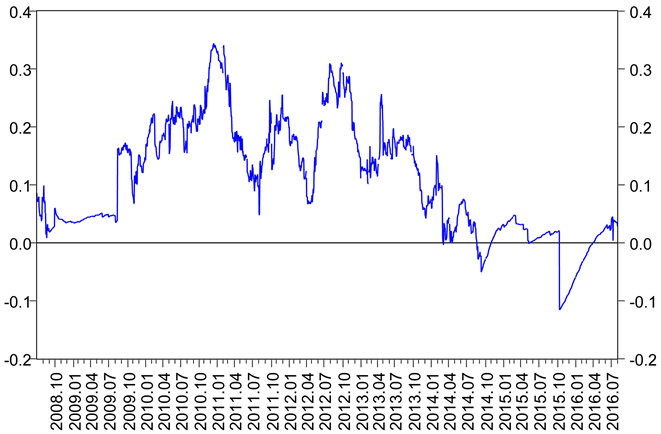Figure 4. Domestic commodity market return and global stock market returns

4. 结论和建议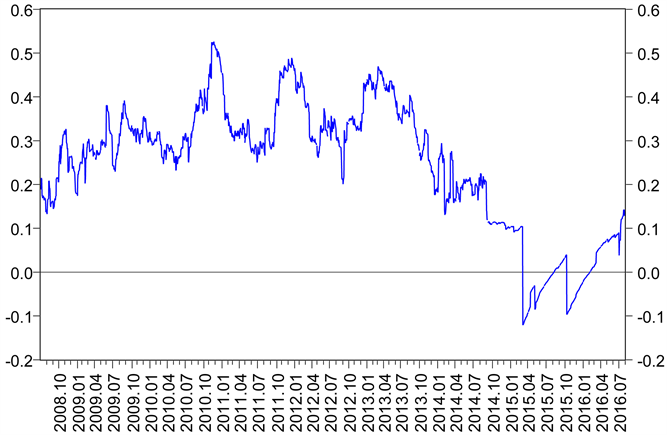Figure 5. Domestic commodity market return and domestic stock market returns

NOTES

*通讯作者。

  Lee, H.S. (2004) International Transmission of Stock Market Movements: A Wavelet Analysis. Applied Economics Letters, 11, 197-201. https://doi.org/10.1080/1350485042000203850  董秀良, 曹凤岐. 国内外股市波动溢出效应——基于多元GARCH模型的实证研究[J]. 数理统计与管理, 2009, 28(6): 1091-1099.  胡金焱, 冯金余. 次债危机对我国股市的跨国风险传染——美国、日本、中国香港与沪深A、B股的证据[J]. 东岳论丛, 2010, 31(2): 42-49.  Gardebroek, C. and Hernandez, M.A. (2012) Do Energy Prices Stimulate Food Price Volatility? Examining Volatility Transmission between US Oil, Ethanol and Corn Markets. Energy Economics, 40, 119-129.  Ewing, B.T. and Malik, F. (2013) Volatility Transmission between Gold and Oil Fu-tures under Structural Breaks. International Review of Economics & Finance, 25, 113-121. https://doi.org/10.1016/j.iref.2012.06.008  徐国祥, 代吉慧. 中国与国际大宗商品市场价格之间的关联性研究[J]. 统计研究, 2015(6): 81-89.  杨胜刚, 成博. 股票市场与大宗商品市场互动特征比较研究[J]. 当代财经, 2014(6): 57-66.  闻岳春, 王婕, 程天笑. 国内股市与国际股市、大宗商品市场的溢出效应研究[J]. 国际金融研究, 2015(8): 31-43.  Beckmann, J. and Czudaj, R. (2014) Volatility Transmission in Agricultural Futures Markets. Economic Modelling, 36, 541-546. https://doi.org/10.1016/j.econmod.2013.09.036  Joseph, A., Sisodia, G. and Tiwari, A.K. (2014) A Frequency Domain Cau-sality Investigation between Futures and Spot Prices of Indian Commodity Markets. Economic Modelling, 40, 250-258. https://doi.org/10.1016/j.econmod.2014.04.019